Courses

# Notes | EduRev

## Class 10 : Notes | EduRev

The document Notes | EduRev is a part of the Class 10 Course Class 10 Mathematics by VP Classes.
All you need of Class 10 at this link: Class 10

NCERT TEXTBOOK QUESTIONS SOLVED

Page No. 311
EXERCISE 15.2

Q 1. Two customers Shyam and Ekta are visiting a particular shop in the same week (Tuesday to Saturday). Each is equally likely to visit the shop on any day as on another day. What is the probability that both will visit the shop on (i) the same day? (ii) consecutive days? (iii) different days?
Sol. Here, the number of all the possible outcomes
= 5 × 5 = 25
(i) For both customers visiting same day:
Number of favourable outcomes = 5
∴ Required probability = 1/25 = 1/5
(ii) For both the customers visiting on consecutive days:
Number of outcomes are: (Tue., Wed.), (Wed., Thu.), (Thu., Fri.), (Fri., Sat.), (Sat., Fri.), (Wed., Tue.), (Thu., Wed.), (Fri., Thu.)
∴ Number of favourable outcomes = 8
⇒ Required probability = 8/25
(iii) For both the customers visiting on different days: We have probability for both visiting same day = 1/5
∴ Probability for both visiting on different days
=1 − [Probability for both visiting on the same day]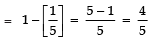⇒ The required probability = 4/5.

Q 2. A die is numbered in such a way that its faces show the numbers 1, 2, 2, 3, 3, 6. It is thrown two times and the total score in two throws is noted. Complete the following table which gives a few values of the total score on the two throws: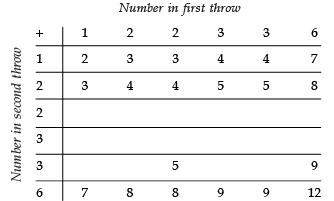What is the probability that the total score is
(i) even? (ii) 6? (iii) at least 6?

Sol. The completed table is as under: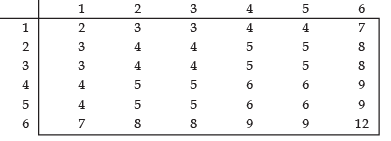∴ Number of all possible outcomes = 36
(i) For total score being even:
Favourable outcomes = 18
[a The even outcomes are: 2, 4, 4, 4, 4, 8, 4, 4, 8, 4, 6, 6, 4, 6, 6, 8, 8]
∴ The required probability = 18/36 = 1/2.
(ii) For the score being 6:
In list of score, we have four 6′ s.
∴ Favourable outcomes = 4
∴ Required probability =  4/36 = 1/9
(iii) For the score being at least 6:
The favourable scores are: 7, 8, 8, 6, 6, 9, 6, 6, 9, 7, 8, 8, 9, 9 and 12
∴ Number of favourable outcomes = 15
⇒ Required probability = 15/36 = 5/12.

Q 3. A bag contains 5 red balls and some blue balls. If the probability of drawing a blue ball is double that of a red ball, determine the number of blue balls in the bag.
Sol. Let the number of blue balls in the bag be x.
∴ Total number of balls = x + 5
Number of possible outcomes = (x + 5).
For a blue ball favourable outcomes = x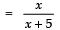Similarly, probability of drawing a red ball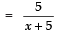Now, we have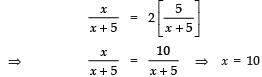Thus the required number of blue balls = 10.

Q 4. A box contains 12 balls out of which x are black. If one ball is drawn at random from the box, what is the probability that it will be a black ball?
If 6 more black balls are put in the box, the probability of drawing a black ball is now double of what it was before. Find x.
Sol. ∵ The total number of balls in the box = 12
∴ Number of possible outcomes = 12
Case-I: For drawing a black ball
Number of favourable outcomes = x
∴ Probability of getting a black ball = x/12.
Case-II: When 6 more black balls are added
Now, the total number of balls
= 12 + 6
= 18
⇒ Number of possible outcomes = 18
Since, the number of black balls now =(x + 6).
⇒ Number of favourable outcomes = (x + 6)
∴ Required probability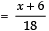Applying the given condition: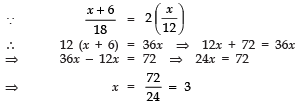Thus, the required value of x is 3.

Page No. 312
Q 5. A jar contains 24 marbles, some are green and others are blue. If a marble is drawn at random from the jar, the probability that it is green is 2/3. Find the number of blue balls in the jar.
Sol. ∵ There are 24 marbles in the jar.
∴ Number of possible outcomes = 24.
Let there are x blue marbles in the jar.
∴ Number of green marbles = 24 − x
⇒ Favourable outcomes = (24 − x)
∴ Required probability for drawing a green marble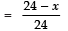Now, according to the condition, we have: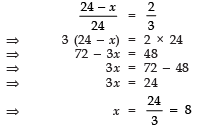Thus, the required number of blue balls is 8.

Offer running on EduRev: Apply code STAYHOME200 to get INR 200 off on our premium plan EduRev Infinity!

132 docs

,

,

,

,

,

,

,

,

,

,

,

,

,

,

,

,

,

,

,

,

,

;